# NCERT Solutions For Class 9 Math Chapter – 7 Exercise – 7.1NCERT Solutions For Class 9 Math Chapter – 7 Exercise – 7.1

#### Experts (adsbygoogle = window.adsbygoogle || []).push({}); at Sunstarup  have created the NCERT Solutions after extensive search on each topic Students can refer to this study study material to boost their confidence and attempt the second term exam smartly. The concepts are explained with steps, shortcuts to remember formula , tips and tricks to solve the numerical problems wisely and quickly.

Q1.   In quadrilateral ABCD  , AC = AD and AB bisects A  Show that ABC   ̴ ∆ABD.  What can you say about BC and BD ?

Solution:-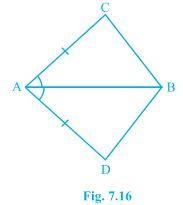AB = AB [Common]

∟CAB = ∟DAB [ AB bisects ∟CAD]

∆ABC ̴ ∆ABD [SAS Rule]

Therefore BC = BD [CPCT]

Q2. ABCD is a quadrilateral in which AD = BC and DAB = CBA (see figure) Prove that

• ABD BAC
• BD = AC
• ABD =

Solution:-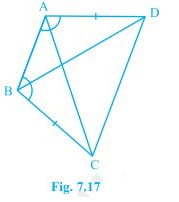• Consider triangles ABD and ABC.

We have AD = BC [Given]

AB = BA [Common]

And

∟DAB = ∟CBA [Given]

∆ABD  ∆BAC

BD = AC

∟ABD = ∟BAC [CPCT]

Q3. AD and BC are equal perpendicular to a line segment AB Show that CD bisects AB.

Solution:-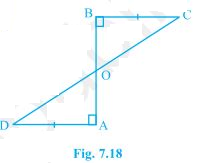AD and BC are perpendicular to AB.

AD // BC and CD is transversal.

OB = OA.

Hence , CD bisects AB.

Q4. l and M are two parallel lines intersected by another pair of parallel lines p and q Show thta ∆ABC  ∆CDA.

Solution:-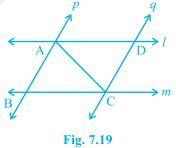∟1 = ∟2 (I) [Alternate angles]

P // Q and AC is transversal

∟3 = ∟4  (ii) (Alternate angles)

AC = CA

∟1 = ∟2 and ∟3 = ∟4

∆ABC  ∆ ABC

AC = CA

∟1 = ∟2  and ∟3 = ∟4

∆ABC  ∆CDA

Q5. Line l is the bisector of an angle ∟A and B is any point on  l BP and BQ  are perpendicular from  to the arms of ∟A Show that:

• ∆APB AQB
• BP = BQ or B is equidistant from the arma of A

Solution:-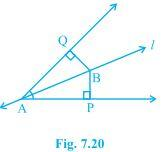Line l is the bisector of ∟QAP.

QAB = ∟PAB.

AB = PA

BQA = ∟BPA

• In triangles AQB and APB.

AB = BA and ∟BQA= ∟BPA

APB  ∆AQB.

• BP = BQ

Q6. In figures AC = AE , AB = AD and ∟BAD = ∟EAC Show that BC = DE.

Solution:-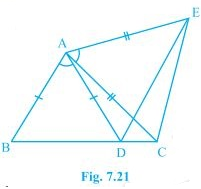∟BAD + ∟DAC = ∟DAC + ∟CAE   [ ∟DAC is added to both sides]

∟BAC = ∟DAE    (i)

In triangles ∆BAC and ∆DAE ,

AC = AE

∟BAC = ∟DAE

∆BAC   ∆DAC

BC = DE.

Q7. AB is a line segment and P is its mid- point D and E are points on the same sides of AB such that BAQ = ABE and EPA = DPB Show that

• ∆DAP EBP

Solution:-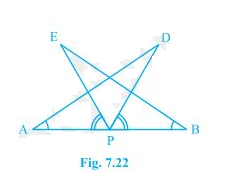∟EPA + ∟EPD = ∟EPD + ∟DPB

∟APD = ∟BPE

AP = BP

∟DAP = ∟EBP

• ∟APD = ∟BPE , AP = BP and ∟DAP = ∟EBP.

∆DAP ∆EBP.

Q8. In right triangle ABC right angled at C , M is the mid point of hypotenuse AB , C is joined to M and produced to a point D such that DM = CM. Point D is joined to point B

Show that:

• AMC BMD
• ∟DBC is a right angle.
• ∆DBC ACB
• CM = 1/2AB.

Solution:-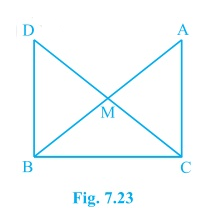• In ∆AMC and DMB ,

∟AMC = ∟BMD (VOA)

∆AMC  ∆BMD [ SAS rule]

• ∟BAC = ∟DBA [CPCT]

AB is the transversal

∟DBC is a right angle

• In ∆ABC and ∆DCB.

AC = DB [Since ∆AMC  ∆BMD ]

BC = CB [Common]

∟ACB = ∟DBC [Each 900]

∆DBC  ∆ACB [SAS rule]

• DC = AB [CPCT from part (iii]

2CM = AB

CM = 1/2AB.

CM  = 1/2AB.